# Must Know HSC Physics Questions for 2018 HSC Physics Exam

Are you ready for 2018 HSC Physics Exam? Make sure you can answer these 18 questions compiled by the Matrix Science team. We've also included detailed solutions to help you get exam ready.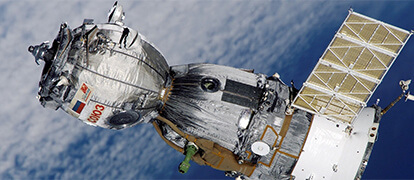2018 HSC Physics Exam is just around the corner. Here are 18 HSC Physics questions the Matrix Science team have recommended for students sitting the 2018 HSC Physics exam.

## Space Module Questions

### Question 1  (6 marks)

Describe how the apparent weight and true weight of an astronaut onboard a space vehicle changes during the following stages of travel:

• Take-off
• Stable orbit
• Re-entry

### Question 2  (5 marks)

Consider a satellite in a stable low earth orbit around the Earth.

(a) Show that the work required to move a satellite from an orbit of radius rto an orbit of radius ris given by:  (3 marks)

$$W = \frac{GMm}{2} (\frac{1}{r_i}- \frac{1}{r_f })$$.

(b) Geostationary satellites are often placed into low Earth orbits first, and then moved to geostationary orbit. Calculate the work required to move a 500 kg satellite from a 350 km altitude orbit to a 35 900 km altitude geostationary orbit. The radius of Earth is 6371 km. (2 marks)

### Question 3  (3 marks)

The diagram shows a spacecraft in an elliptical orbit around the Sun.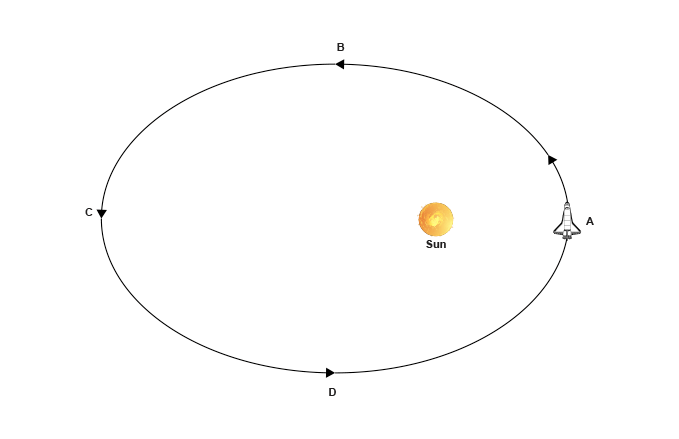(a) Identify the position (A, B, C or D) at which the spacecraft has greatest kinetic energy. (1 mark)

(b) With reference to the law of conservation of energy, explain how the velocity of the rocket changes as it orbits the sun in an elliptical orbit from position A to C.  (3 marks)

### Question 4  (4 marks)

The graph of distance cubed against period squared for the four largest moons of Jupiter (Io, Europa, Ganymede and Callistor) are shown below. Each moon has a period denoted by T and a distance from Jupiter’s centre denoted by R.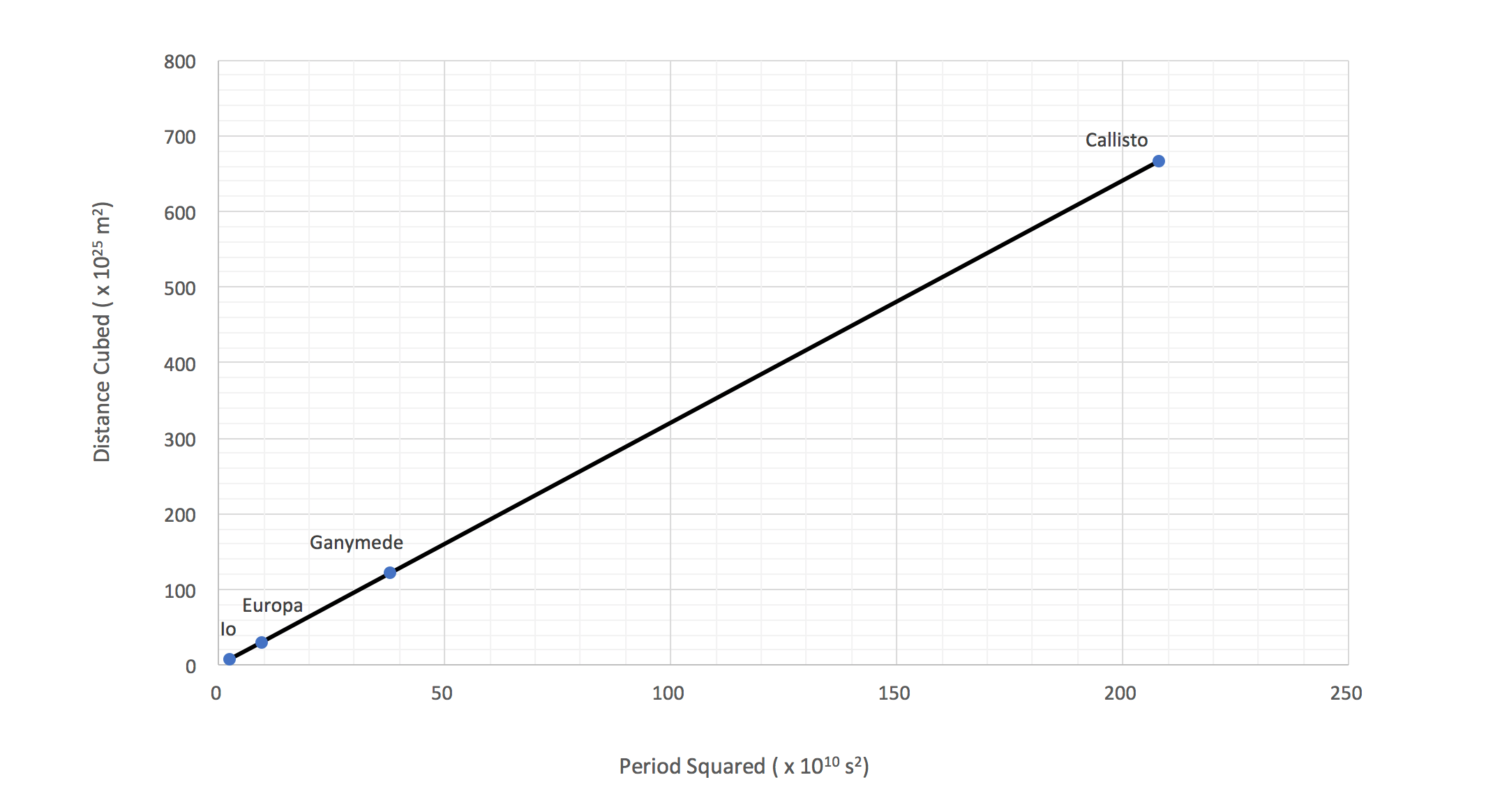(a) Explain how the graph shows that Kepler’s third law applies to the moons of Jupiter. (2 marks)

(b) From the graph, determine the mass M of Jupiter. (2 marks)

### Question 5  (4 marks)

Consider the following statement made by a Physicist:

‘It is possible to use the slingshot effect to increase the velocity of a spacecraft’.

Explain how the statement is true in some frames of reference but not in others.  (3 marks)

## Need help acing your Physics exam?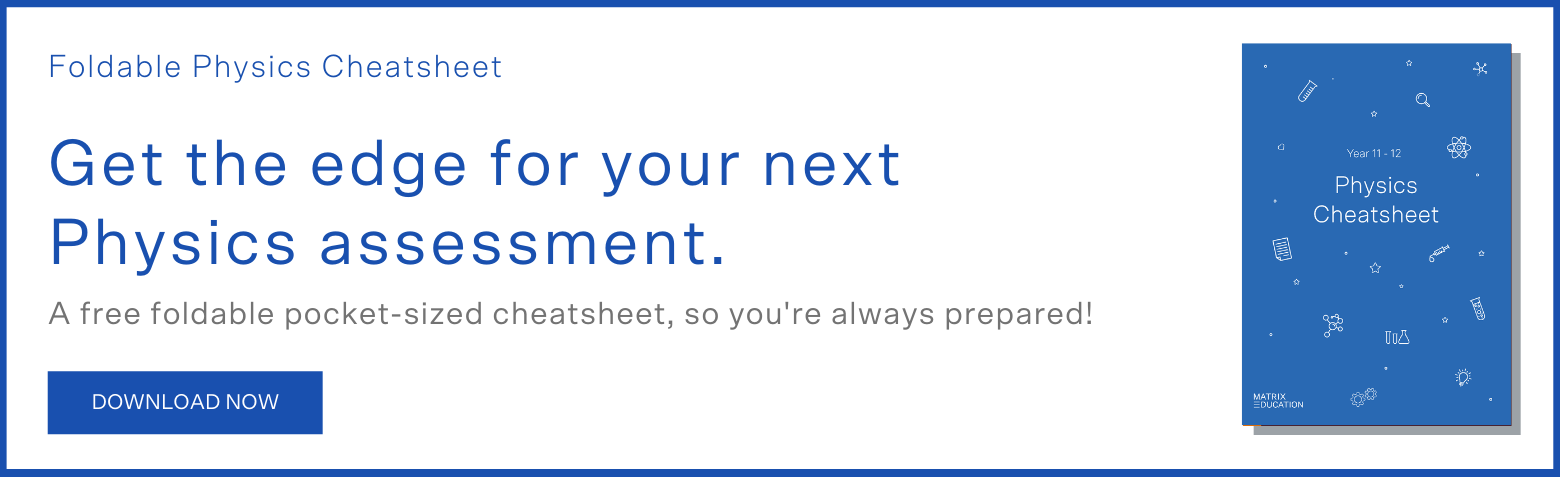Motors and Generators Module Questions

### Question 6  (4 marks)

Three long wires X, Y, Z are placed parallel to each other as shown below.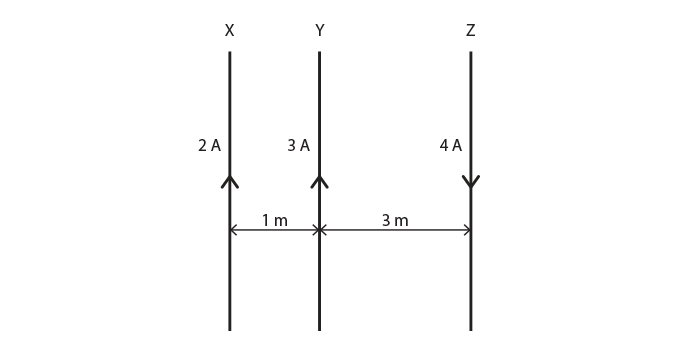Determine the magnitude and direction of the resultant force per unit length acting on wire X.

### Question 7  (7 marks)

An old bicycle design is a penny-farthing with a large front wheel to which pedals are directly attached as shown in the diagram below.The large front wheel has a 66 cm radius, and the pedals extend 15 cm from the axle. The mass of the bicycle is 13.6 kg.

Calculate:

(a) the maximum torque a 70 kg rider can apply to the pedals by standing on one. (2 marks)

(b) the maximum acceleration of the bicycle and rider from the torque applied in (a). (3 marks)

(c) the maximum uphill angle (to the nearest degree) that the rider can ride this bicycle. (2 marks)

### Question 8   (3 marks)

A diagram of a galvanometer is shown.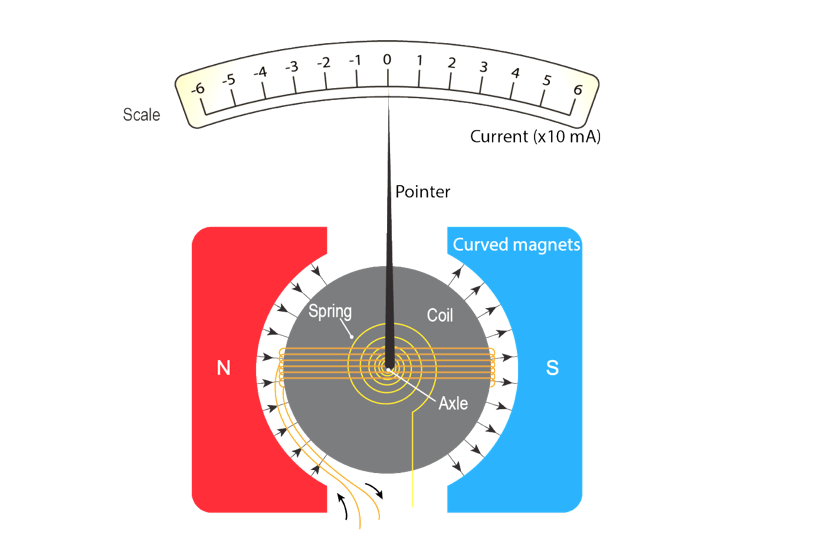Explain the effect of replacing radial magnets with plane magnets to the operation of a galvanometer.

### Question 9   (3 marks)

A square coil, ABCD is moving at constant velocity v, at right angles to a uniform magnetic field B as shown.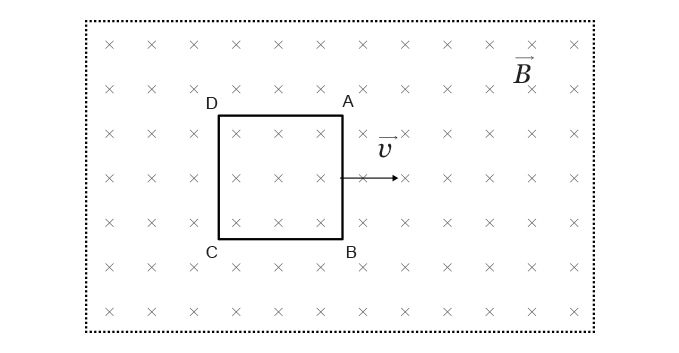(a) Which side of the square coil (AB, BC, CD, or DA) has the greatest concentration of electrons?

(b) Is the overall induced EMF in the coil maximum or minimum? Give a reason for your answer.

### Question 10   (3 marks)

The graph shows the change in torque for a DC motor from startup to operating speed.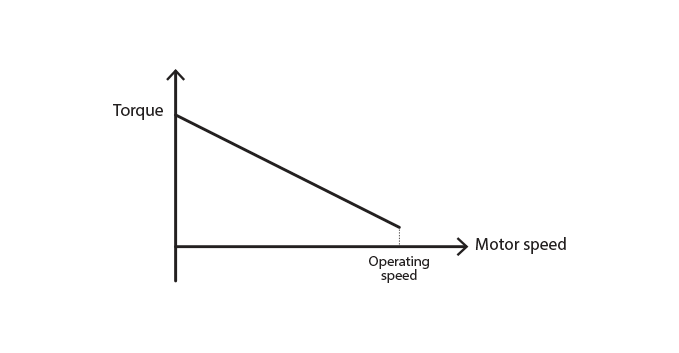Explain why the torque in a DC motor varies with its speed.

### Question 11   (3 marks)

A diagram shows a simple AC generator.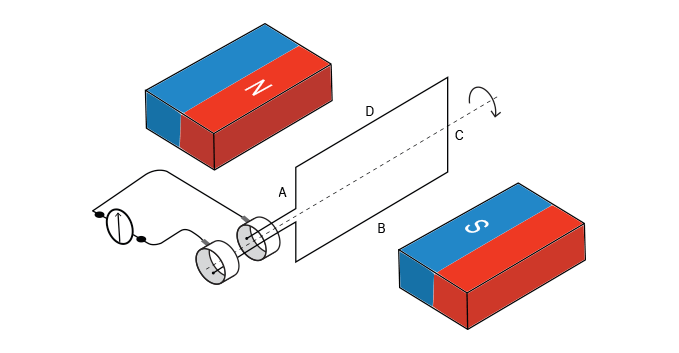(a) Sketch a graph of induced EMF vs time for one rotation.

(b) Describe three changes that will maximise the EMF induced by the AC generator.

### Question 12   (3 marks)

Electrical power is generated in a power plant and is transmitted to your house through a system called the power distribution grid.

In the distribution of electricity, the overall energy losses between the power plant and the users can easily be between 8-15%. This suggest there is still room to improve efficiency.

(a) Explain the existing sources of electrical power loss.  (3 marks)

(b) Suggest a possible new technology to minimise the electrical power loss.  (2 marks)

## From Ideas to Implementation Module Questions

### Question 13   (7 marks)

An electron is projected horizontally into the uniform electrostatic field between horizontally placed parallel plates with a velocity of 1.0 x 10m/s. The space between the plates has been evacuated. The charge of the electron is -1.602 x 10-19  C and the mass of the electron is 9.109 x10-31 kg.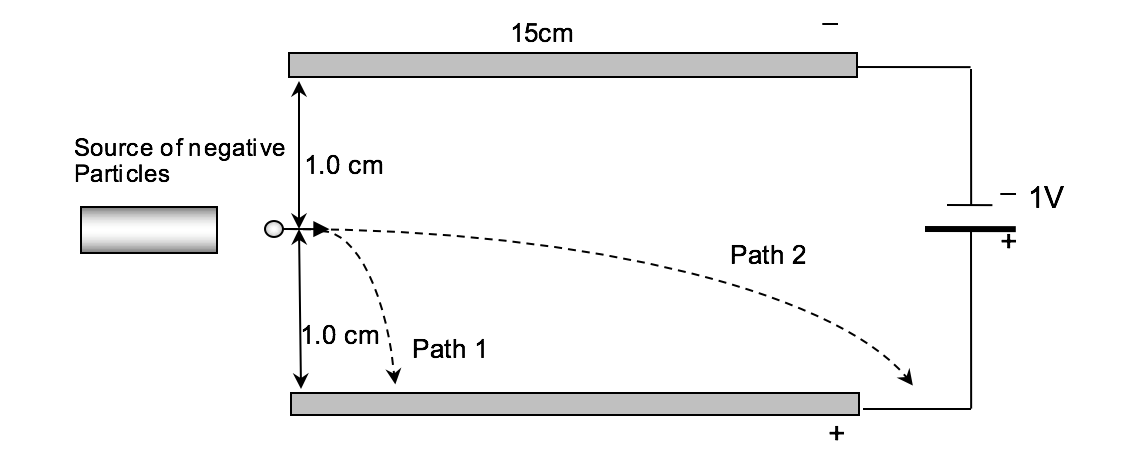(a) Which path will the electron follow? Support your answer with appropriate calculations.  (4 marks)

(b) A magnetic field is applied so that electrons travel undeflected. Calculate the magnitude and direction of the magnetic field.  (3 marks)

### Question 14   (3 marks)

Describe how Hertz experimentally calculated the speed of the radio waves he generated and detected. Include any relevant diagrams.

### Question 15   (4 marks)

Discuss Maxwell and Planck’s view of electromagnetic radiation in terms of

• fundamental mechanism for production of electromagnetic radiation

### Question 16   (7 marks)

(a) State Einstein’s Photon Theory of Light.  (2 marks)

(b) With reference to the photon theory of light, explain the statement, ‘light is quantised’. (2 marks)

(c) Describe the results of an experiment that supports Einstein’s Photon Theory of light. (3 marks)

### Question 17   (7 marks)

A p-n junction is a simple 2-layer device formed by sticking a piece of p-type semiconductor to a piece of n-type semiconductor.

(a) Draw the band structure of a p-n junction. Clearly label the valence band, conduction band and dopant levels. (3 marks)

(b) Describe the process that leads to the unique electrical property of a p-n junction.  (3 marks)

(c) State one application of p-n junctions (1 mark)

### Question 18   (4 marks)

The diagram below shows two identical materials at two different temperatures.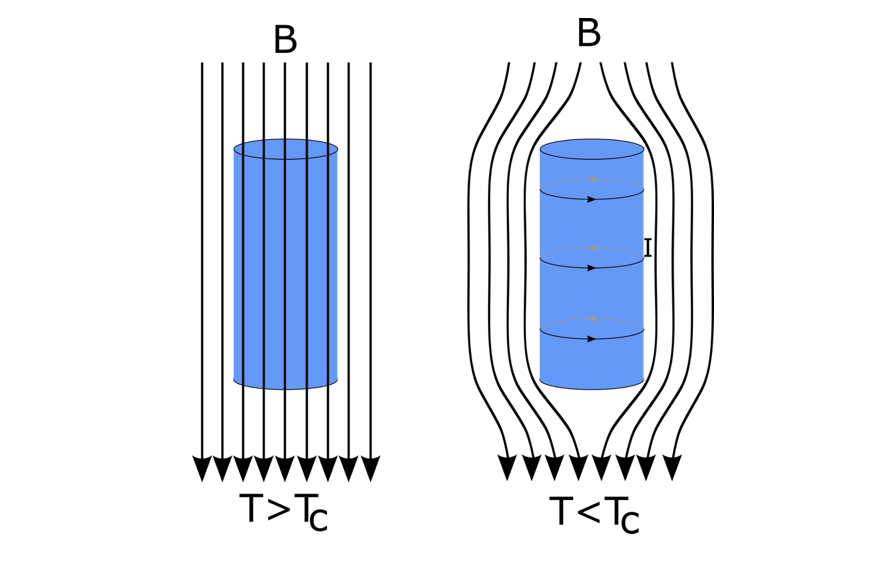The material on the left is at a temperature above critical temperature and the material on the right is at a temperature below critical temperature.

(a) State the phenomenon the diagram illustrates.  (1 mark)

(b) Explain why the external magnetic fields do not penetrate the material on the right.  (3 marks)

Question 1

 Stage Apparent weight vs True Weight Take-off Apparent weight is greater than true weight (mg) as the acceleration of the space vehicle is upwards.(Note: True weight decreases as the altitude increases due to the decrease in gravitational fields strength.) Stable Orbit Apparent weight is zero as it is ‘falling’ at the same rate as the space vehicle. True weight (mg) in orbit is smaller than on earth since the gravitational field strength is smaller. Re-entry Apparent weight is greater than true weight (mg) as the the acceleration of the space vehicle is upwards due to increasing atmospheric drag.(Note: True weight increases as the altitude decreases and the gravitational field strength increases.)

Question 2

 (a) Work = Change in KE + Change in GPE(Note that both kinetic and potential energy change in the transfer of orbits) $$W =\frac{1}{2}[(v_f)^{2} – (v_i)^{2}] + [-GMm (\frac{1}{r_f}- \frac{1}{r_i})]$$ $$W =\frac{1}{2}[(\sqrt{\frac{GM}{r_f}})^{2} – (\sqrt{\frac{GM}{r_i}})^{2}] + [-GMm (\frac{1}{r_f}- \frac{1}{r_i})]$$ $$W =\frac{1}{2}[\frac{GM}{r_f} – \frac{GM}{r_i}] + [\frac{-2GMm}{2} (\frac{1}{r_f}- \frac{1}{r_i })]$$ $$W =[\frac{-GMm}{2} (\frac{1}{r_f}- \frac{1}{r_i })]$$ $$W =[\frac{+GMm}{2} (\frac{1}{r_i}- \frac{1}{r_f })]$$ (b) $$W =[\frac{+GMm}{2} (\frac{1}{6371000+350000}- \frac{1}{6371000+35900000})]$$ $$W=1.25 \times 10^{10} J$$

Question 3

 (a) Position A.The distance from the sun is smallest and therefore its gravitational potential energy is the smallest. Hence its kinetic is the greatest. . (b) According to the law of conservation of energy: The total energy of a system must remain constant.As the rocket moves from A to C, its distance from the sun increases.Therefore, its gravitational potential energy increases since it increases with increasing radius of orbit and its kinetic energy decreases.The increase in gravitational potential energy equal to the decrease in kinetic energy.Hence the velocity of the rocket decreases as it moves from position A to C.

Question 4

 (a) Period squared is directly proportional to distance cubed.This implies the ratio of period squared and distance cubed is constant as per Kepler’s Third Law. (b) $$T^2=\frac{4\pi^2}{GM} R^3$$ $$Gradient =\frac{4\pi^2}{GM}$$ $$M =\frac{4\pi^2}{G \times Gradient}$$ $$W=1.9 \times 10^{27} J$$

Question 5

 Relative to the Planet Change in speed The spacecraft’s velocity relative to the planet is unchanged as its exit speed is equal to entry speed. Law of Physics Gravitational force of attraction accelerates and decelerates the spacecraft during entry and exit.

 Relative to the Sun Change in speed The spacecraft’s velocity relative to the Sun is changed (usually increased). Law of Physics There was a transfer of momentum from the planet to the spacecraft.

Question 6

Force per unit length between wires X and Y is attractive. Therefore the force on the wire X due to wire Y is to the right.

$$\frac{F}{L}=\frac{KI_x I_y}{d}=\frac{2 \times 10^{-7} \times 2 \times 3}{1}=1.2 \times 10^{-6}$$ N/m to the right

Force per unit length between wires X and Z is repulsive. Therefore the force on the wire X due to wire Z is to the left.

$$\frac{F}{L}=\frac{KI_x I_y}{d}=\frac{2 \times 10^{-7} \times 2 \times 4}{4}=4 \times 10^{-7}$$ N/m to the left

Resultant force per unit length on wire X:

$$\frac{F}{L}=1.2 \times 10^{-6} right + 4 \times 10^{-7} left =8 \times 10^{-7}$$ N/m to the right

Question 7

 (a) $$T = F \times r$$ $$T = mg \times r$$ $$T = 70 \times 9.8 \times 0.15$$ $$T = 102.9 \text{Nm}$$ Nm (b) Since the pedal and the wheel share the same axis of rotation, the torque applied to the pedal equals to the torque experience by the wheel. $$T_{pedal}=T_{wheel}$$$$102.9 = F \times 0.66$$ $$F =\frac{102.9}{0.66}=155.91N$$ $$a=\frac{F}{m}=\frac{155.91}{70+13.6} = 1.86 m/s^{2}$$ (c) $$F_{net}=ma$$ $$mg sin\theta=ma$$$$a=g sin\theta$$ $$1.86=9.8 sin\theta$$ $$sin\theta = 0.190$$ $$\theta = 11 ^\circ$$

Question 8

When the radial magnets are replaced with plane magnets:

• the plane of the coil will no longer be always parallel to the magnetic field
• the torque produced by the coil will no longer be a maximum constant value
• the scale will no longer be linear

Question 9

 (a) BC. (b) Minimum.The induced EMF across the conductor DC and AB oppose each other with equal magnitude and hence the overall EMF is equal to zero.ORThe change in magnetic flux is zero.

Question 10

 When the coil is stationary There is no change in flux experienced by the coil and hence back EMF is zero (Faraday’s law). As a result, the net EMF in the coil is equal to the supply EMF only and therefore the current in the coil is maximum. Hence torque is also maximum ($$\tau = nBIA$$). When the coil starts to rotate The rate of change in flux increases and hence back EMF also increases. As a result, the net EMF in the coil ($$EMF_{net} = EMF_{supply} – EMF_{back}$$) and current coil decreases. Hence torque decreases steadily as the coil spins faster. When the coil is rotating at its operating speed The rate of change in flux is maximum and hence back EMF is also maximum. As a result, the net EMF in the coil is minimum (zero in an ideal motor). Hence torque is minimum at its operating speed.

Question 11

 (a)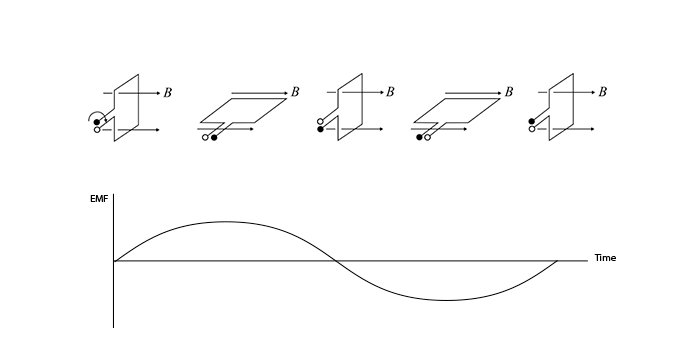(b) Increase the speed of rotationIncrease the number of coilsIncrease the magnetic field strength

Question 12

 (a) There are two main areas for power loss in transmission.In step down transformers, a large current flows in the secondary windings after voltage has been decreased. Hence a large energy loss takes place in the secondary windings due to resistive heating.Transmission lines: Power loss in transmission lines occurs due to resistive heating in the wires. During the transmission near the generator the power loss quite small since the current is usually low. Step down transformers are transmitting at a higher voltages and low currents. Closer  to the households, voltage is stepped down and hence higher currents flow through the transmission lines. This results in higher power loss. (b) Superconductors have zero resistance and therefore incur no power loss when used as transmission wires.

Question 13

 (a) $$a=\frac{F_{net}}{m}=\frac{Eq}{m} \\E=\frac{V}{d}=\frac{1}{0.02}=50 V/m$$ $$a=\frac{50 \times 1.602 \times 10^{-19}}{9.109 \times 10^{-31}}= 8.8 \times 10^{12}t^{2}$$  $$y=ut + \frac{1}{2} at^{2}$$  $$-0.01=0+\frac{1}{2} \times 8.8 \times 10^{12}t^{2}$$  $$t=4.77 \times 10^{-9} s$$ Horizontal Distance Travelled $$x=u_x \times t = 1.0 \times 10^{6} \times 4.77 \times 10^{-9} = 0.0476 m = 4.76 cm$$ Hence it follows path 1. (b) $$qE = qvB$$$$E = vB$$ $$50 = 1.0 \times 10^{6} \times B$$ $$B = \frac {50}{1.0 \times 10^{6}} = 5 \times 10^{-5}$$ TThe direction of magnetic field is perpendicular to the page and out of the page.

Question 14

In order to calculate the speed of the waves, you require the frequency and wavelength of the waves since $$v = f \lambda$$

 Frequency Hertz calculated the frequency of the transmitter (and the EM wave) from the known parameters of the circuit. Wavelength Hertz then formed a standing wave of electromagnetic radiation by reflecting the radio waves from a large flat zinc plate.Hertz moved his receiver coil along this wave. A spark in the gap was produced at the anti-nodes (constructive interference) but not at the nodes (destructive interference). The distance travelled between nodes or anti-nodes is half a wavelength. Doubling this distance gave the wavelength. Speed Using the wave equation, $$v = f \lambda$$ he calculated the speed of the waves as  as predicted by Maxwell.

Question 15

 Maxwell Planck Fundamental Mechanism for Production of Electromagnetic Radiation Acceleration of charged particles.Whenever a charged particle undergoes acceleration, it must release energy in the form of electromagnetic waves.The energy of the electromagnetic waves is continuous and is carried evenly distributed across the wavefronts. A change in quantum state.Whenever an oscillating atom or molecule undergoes a change in quantum state, it releases discrete energy in the form of electromagnetic waves.Hence the energy of the electromagnetic waves is emitted and absorbed in discrete packets of energy.The amount of energy carried by the packet determines the frequency of electromagnetic wave according to the equation, E = hf Nature of Electromagnetic Radiation Electromagnetic waves consist of oscillations of electric and magnetic fields, which are at right angles to each other and also at right angles to the direction of wave propagation. Electromagnetic waves consist of oscillations of electric and magnetic fields, which are at right angles to each other and also at right angles to the direction of wave propagation.

Question 16

 (a) light(or any electromagnetic wave) is a stream of particles known as photons.Each photon has an energy, given by: E = hf (b) The energy of a photon is the smallest amount of energy electromagnetic radiation can carry and hence is an indivisible unit of electromagnetic energy. Therefore the electromagnetic energy absorbed must be in whole number multiples of the photon energy. Hence the light energy is quantised. (c) No electrons are emitted if the incident light frequency falls below some threshold or cut-off frequency, , which is characteristic of the material being illuminated.If the light frequency exceeds the cut-off frequency, a photoelectric effect is observed and the number of photoelectrons emitted is proportional to the light intensityThe maximum kinetic energy of the photoelectrons is independent of light intensity. The maximum kinetic energy of the photoelectrons increases with increasing light frequency.Electrons are emitted from the surface almost instantaneously (less than 10-9seconds after the surface is illuminated), even at low light intensities.

Question 17

 (a)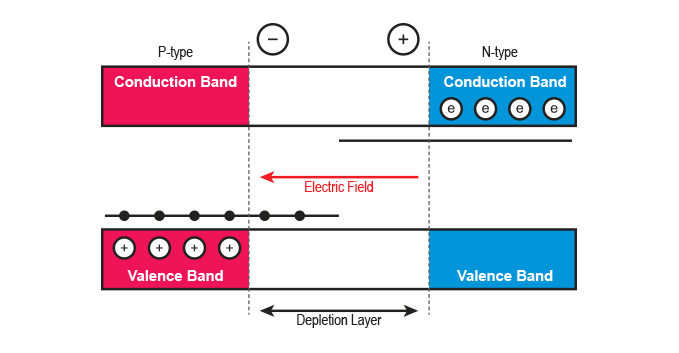(b) Since the n-type semiconductor has free electrons and the p-type semiconductor has holes, electrons move to fill the holes.The movement of electrons from the n-type semiconductor to the p-type semiconductor gives rise to the depletion region or depletion layer.As the n-type layer lost electrons it becomes positively charged. The p-type layer gained electrons and becomes negatively charged. Hence, an electric field is created across the depletion layer. (c) solar cells

Question 18

 (a) The expulsion of a magnetic field from the interior of a superconductor is known as the Meissner effect. (b) When its temperature is greater than the critical temperature, the magnetic field penetrates the cylinder.When the temperature is lowered below the critical temperature, the magnetic field is spontaneously expelled from the interior of the superconductor as shown on the right. In this case, surface currents (consisting of copper pairs) are induced on the superconductor, producing a magnetic field which exactly cancels the externally applied field inside the superconductor.